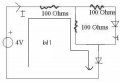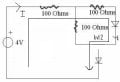# Solving Simple Circuit, Diodes Parallel (part 2)

Thread Starter

#### Student01

Joined Apr 15, 2009
35
Hey guys,

I'm sorry but I have no idea where to begin on this question, could I please get a step-by-step run-through of how you approach this? I have in the text book a simpler example, but it doesn't have diodes in parallel (with resistors) and I don't understand this "assume diode is off and test for consistency" method it describes.

Find I, assume voltage across forward-biased diode is 0.7 V#### italo

Joined Nov 20, 2005
205
use thevenin analysis solve for the first circuit and add that in series to the other. .6,.7 is an approximation of a diode forward conducting since it is an exponetial curve related to current Vs volts.

#### kiranag

Joined Apr 14, 2009
12
First apply KVL to the branch shown. The branch including source, 100ohm resistor, and the part of the parallel branch which excludes the diode. U can easily find I(current through the main circuit)Then apply second KVL equation to this path to find the required current flowing through the branch.Simple. Take the voltage across the diodes as 0.7v while tracing the branches for KVL. Hope you got it.

#### PRS

Joined Aug 24, 2008
989
Here's how I think it should be solved. Good luck with it!Thread Starter

#### Student01

Joined Apr 15, 2009
35
Thanks for the replies guys, but I was hoping for a bit more of an indepth walk-through. This is something I'm really struggling with and find it difficult to fill in the blanks, even if they may seem obvious. I don't need instructions on how to solve, so much, but an approach to the question and why each step is taken in solving.

Does anyone have any links to walk-throughs for similar questions? I've tried googling, but it's difficult to know what search terms to use. So far I've come up with nothing.

italo, I'm afraid I don't understand much of anything you said. I need a much slower, more in-depth explanation.

kiranag, your explanation was good, but I need to know how and why you do each step.

PRS, I don't understand where you got the "By Inspection" part of your solution.

#### DrNick

Joined Dec 13, 2006
110
There are two diodes in series. Assuming these are ideal "silicon" diodes with a forward voltage of 0.7V, if any current is flowing through them at all there will be 0.7V drop. 2 x 0.7 = 1.4. So "by inspection" there are two diodes both with combined a 1.4V drop.

Thread Starter

#### Student01

Joined Apr 15, 2009
35
Thanks, DrNick.

From what I understand, PRS has used NTD Analysis. Could someone please show me an alternative way of solving, using KVL, KCL, or any other method. I need to learn how to approach questions like this and be able to select the easiest method of solving. I'd like to be able to compare.

#### DrNick

Joined Dec 13, 2006
110
One thing that is handy is, if you have a diode in series with a power supply (in either the positive or negative terminal). In this case there is one in series with the negaitve lead. What you can do is just remve the second diode from the circuit and subtract .7V from the power supply voltage.

After you do that the voltage souce becomes 3.3V and now you just have the one diode to deal with in the circuit. This may make the circuit look more familiar to you.

Thread Starter

#### Student01

Joined Apr 15, 2009
35
Does this work in all case, DrNick? Even when the diode is "off"? (I still don't completely understand the concept of how you can tell if a diode is on or not.)

Do you add the 0.7 V if the diode is in series with the positive terminal?

#### DrNick

Joined Dec 13, 2006
110
it does not work when the diode is off. this only works in the insance that the diode conducts and is in series with the power supply. you can not just place a diode in front of the power supply in any circuit. It has to be there already.

Thread Starter

#### Student01

Joined Apr 15, 2009
35
it does not work when the diode is off.
How do I tell if the diode is on?

this only works in the insance that the diode conducts and is in series with the power supply.
Do I always subtract from the voltage source, regardless of which terminal the diode is in series with?

you can not just place a diode in front of the power supply in any circuit. It has to be there already.
I don't understand. The diode is there because the circuit diagram states it is.

#### kiranag

Joined Apr 14, 2009
12
Ya. I think i have an answer for that question. First of all, while solving any problem pertaining to analysis of circuits, u need to note down the values of every parameters. For ex, while solving for current through a resistor, u have to get the values of voltage across the resistor and the value of resistance so that u wil get the current flowing through it by dividing one by other. KVL and KCL are the two basic laws which are useful to just calculate the value of nth current or voltage given n-1 values. Here, by KVL 1, u will get the value of the main circuit current since all the parameters related to that loop are known leaving that I as unknown. By using the value of I and by applying KVL 2, u can find out another current flowing through the diode of parallel branch since this is the only unknown parameter of the loop. Satisfactory? If not, please let me know. Thank you.

Thread Starter

#### Student01

Joined Apr 15, 2009
35
This explains a lot, thanks, kiranag. However I get two unknowns when applying "KVL 1": the current through the top node (through the 100Ω in series with the 4V) and the current through 100Ω in parallel with the third 100Ω and diode.

This is my approach:

KVL
-4 + 100I(1) + 100I(2) + 0.7 = 0

Where I(1) and I(2) are the two currents.

#### PRS

Joined Aug 24, 2008
989
Hello again, Student01. I'm sorry for not explaining the solution. You're right -- what is obvious to me may not be, and probably isn't that obviouls to a beginning student. DrNick is right about .7 volts across each diode and adding up 2 diodes in series gives 1.4 volts. (The voltage drop is caused by the diode's internal resistance and ohms law with regard to the current flowing through it. For silicon this drop is approximately .6 to.7 volts. For germanium it is approximately .2 volts).

Once you have determined these diode-created voltages, you should think 'what do I need to do to find i? A little thought suggests ohms law across the 100 ohm resistor in the same branch of the circuit as i. But we don't know v at that central node. If we did we'd have our solution.

It will be, with experience, 'obvious' that KCL should be used at that node. Not loop equations using KVL. One node equation in the first case and two loop equations in the second case. Keep it simple.

The KCL node equation is made simple by the fact that we know all the voltages and resistances leading into that node, hence one equation will do.

What you need is practice. And if you want an aid in this there is a book at your university book store called Problem Solver: Electric Circuits. It give you solutions to many problems typically encountered by the student.

Good luck!Thread Starter

#### Student01

Joined Apr 15, 2009
35
Thanks, PRS. I think I've got about as much use from this thread as I can.

Similar threads